# 1-dimensional hard rods

1-dimensional hard rods are basically hard spheres confined to 1 dimension (not to be confused with 3-dimensional hard rods). The model is given by the intermolecular pair potential: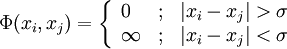$\Phi (x_i,x_j) = \left\{ \begin{array}{lll} 0 & ; & |x_i-x_j| > \sigma \\ \infty &; & |x_i-x_j| < \sigma \end{array} \right.$

where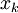$\left. x_k \right.$ is the position of the center of the k-th rod, along with an external potential; the whole length of the rod must be inside the range: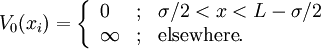$V_{0}(x_i) = \left\{ \begin{array}{lll} 0 & ; & \sigma/2 < x < L - \sigma/2 \\ \infty &; & {\mathrm {elsewhere}}. \end{array} \right.$

## Canonical Ensemble: Configuration Integral

The statistical mechanics of this system can be solved exactly (see Ref. 1). Consider a system of length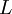$\left. L \right.$ defined in the range$\left[ 0, L \right]$. The aim is to compute the partition function of a system of$\left. N \right.$ hard rods of length$\left. \sigma \right.$. Consider that the particles are ordered according to their label: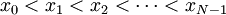$x_0 < x_1 < x_2 < \cdots < x_{N-1}$; taking into account the pair potential we can write the canonical partition function (configuration integral) of a system of$N$ particles as: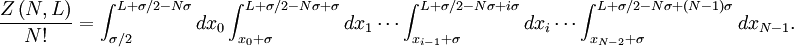$\frac{ Z \left( N,L \right)}{N!} = \int_{\sigma/2}^{L+\sigma/2-N\sigma} d x_0 \int_{x_0+\sigma}^{L+\sigma/2-N\sigma+\sigma} d x_1 \cdots \int_{x_{i-1}+\sigma}^{L+\sigma/2-N\sigma+i \sigma} d x_i \cdots \int_{x_{N-2}+\sigma}^{L+\sigma/2-N\sigma+(N-1)\sigma} d x_{N-1}.$

Variable change: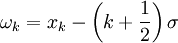$\left. \omega_k = x_k - \left(k+\frac{1}{2}\right) \sigma \right.$ ; we get: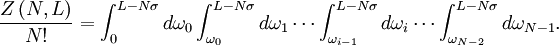$\frac{ Z \left( N,L \right)}{N!} = \int_{0}^{L-N\sigma} d \omega_0 \int_{\omega_0}^{L-N\sigma} d \omega_1 \cdots \int_{\omega_{i-1}}^{L-N\sigma} d \omega_i \cdots \int_{\omega_{N-2}}^{L-N\sigma} d \omega_{N-1}.$

Therefore: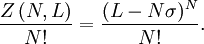$\frac{ Z \left( N,L \right)}{N!} = \frac{ (L-N\sigma )^{N} }{N!}.$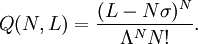$Q(N,L) = \frac{ (L-N \sigma )^N}{\Lambda^N N!}.$

## Thermodynamics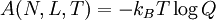$\left. A(N,L,T) = - k_B T \log Q \right.$

In the thermodynamic limit (i.e.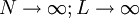$N \rightarrow \infty; L \rightarrow \infty$ with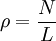$\rho = \frac{N}{L}$, remaining finite):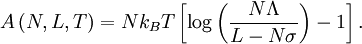$A \left( N,L,T \right) = N k_B T \left[ \log \left( \frac{ N \Lambda} { L - N \sigma }\right) - 1 \right].$

## Equation of state

Using the thermodynamic relations, the pressure (linear tension in this case)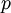$\left. p \right.$ can be written as: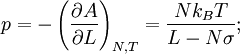$p = - \left( \frac{ \partial A}{\partial L} \right)_{N,T} = \frac{ N k_B T}{L - N \sigma};$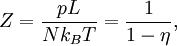$Z = \frac{p L}{N k_B T} = \frac{1}{ 1 - \eta},$

where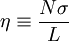$\eta \equiv \frac{ N \sigma}{L}$; is the fraction of volume (i.e. length) occupied by the rods.

## Isobaric ensemble: an alternative derivation

Adapted from Reference . If the rods are ordered according to their label:$x_0 < x_1 < x_2 < \cdots < x_{N-1}$ the canonical partition function can also be written as: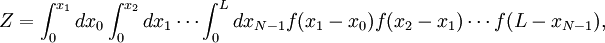$Z= \int_0^{x_1} d x_0 \int_0^{x_2} d x_1 \cdots \int_0^{L} d x_{N-1} f(x_1-x_0) f(x_2-x_1) \cdots f(L-x_{N-1}),$

where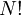$N!$ does not appear one would have$N!$ analogous expressions by permuting the label of the (distinguishable) rods.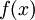$f(x)$ is the Boltzmann factor of the hard rods, which is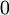$0$ if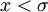$x<\sigma$ and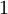$1$ otherwise.

A variable change to the distances between rods: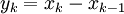$y_k = x_k - x_{k-1}$ results in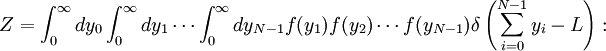$Z = \int_0^{\infty} d y_0 \int_0^{\infty} d y_1 \cdots \int_0^{\infty} d y_{N-1} f(y_1) f(y_2) \cdots f(y_{N-1}) \delta \left( \sum_{i=0}^{N-1} y_i-L \right):$

the distances can take any value as long as they are not below$\sigma$ (as enforced by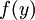$f(y)$) and as long as they add up to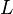$L$ (as enforced by the Dirac delta). Writing the later as the inverse Laplace transform of an exponential: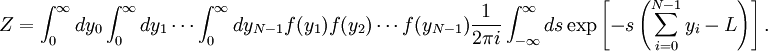$Z = \int_0^{\infty} d y_0 \int_0^{\infty} d y_1 \cdots \int_0^{\infty} d y_{N-1} f(y_1) f(y_2) \cdots f(y_{N-1}) \frac{1}{2\pi i } \int_{-\infty}^{\infty} ds \exp \left[ - s \left(\sum_{i=0}^{N-1} y_i-L \right)\right].$

Exchanging integrals and expanding the exponential the$N$ integrals decouple: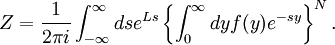$Z = \frac{1}{2\pi i } \int_{-\infty}^{\infty} ds e^{ L s } \left\{ \int_0^{\infty} d y f(y) e^{ - s y } \right\}^N.$

We may proceed to invert the Laplace transform (e.g. by means of the residues theorem), but this is not needed: we see our configuration integral is the inverse Laplace transform of another one,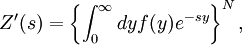$Z'(s)= \left\{ \int_0^{\infty} d y f(y) e^{ - s y } \right\}^N,$

so that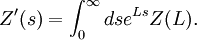$Z'(s) = \int_0^{\infty} ds e^{ L s } Z(L).$

This is precisely the transformation from the configuration integral in the canonical (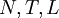$N,T,L$) ensemble to the isobaric (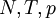$N,T,p$) one, if one identifies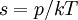$s=p/k T$. Therefore, the Gibbs energy function is simply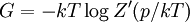$G=-kT\log Z'(p/kT)$, which easily evaluated to be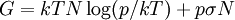$G=kT N \log(p/kT)+p\sigma N$. The chemical potential is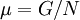$\mu=G/N$, and by means of thermodynamic identities such as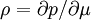$\rho=\partial p/\partial \mu$ one arrives at the same equation of state as the one given above.